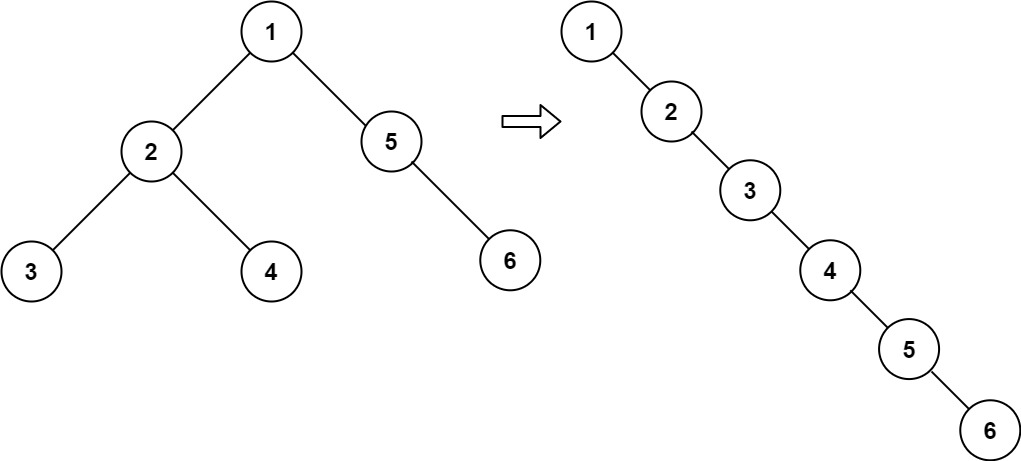# Leetcode 114 - Flatten binary tree to linked list

Note:

• Use recursion with O(1) extra space.
• Flatten left subtree first.
• Let root.right = left.
• Use tmp to get the last node of flattened left.
• Let tmp.next = right.

Question:

Given the root of a binary tree, flatten the tree into a “linked list”:

• The “linked list” should use the same TreeNode class where the right child pointer points to the next node in the list and the left child pointer is always null.
• The “linked list” should be in the same order as a pre-order traversal of the binary tree.

Example:Code: